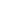Flat 50% off

Ends in

• JEE Main & Advanced
•• 11th
•• Physics
• Back to Doubt Clearing

## Gas expanding adiabatically to temperature $T_2$. If $L_1$ and $L_2$ are lengths of the gas column before and after expansion respectively, then $\frac {T_1}{T_2}$ is given by (a) $(\frac {L_1}{L_2})^\frac 23$ (b) $(\frac {L_1}{L_2})$ (c) $(\frac {L_2}{L_1})$ (d) $(\frac {L_2}{L_1})^\frac 23$

### Asked By Pratibha

Updated Mon, 13 Aug 2018 10:13 am